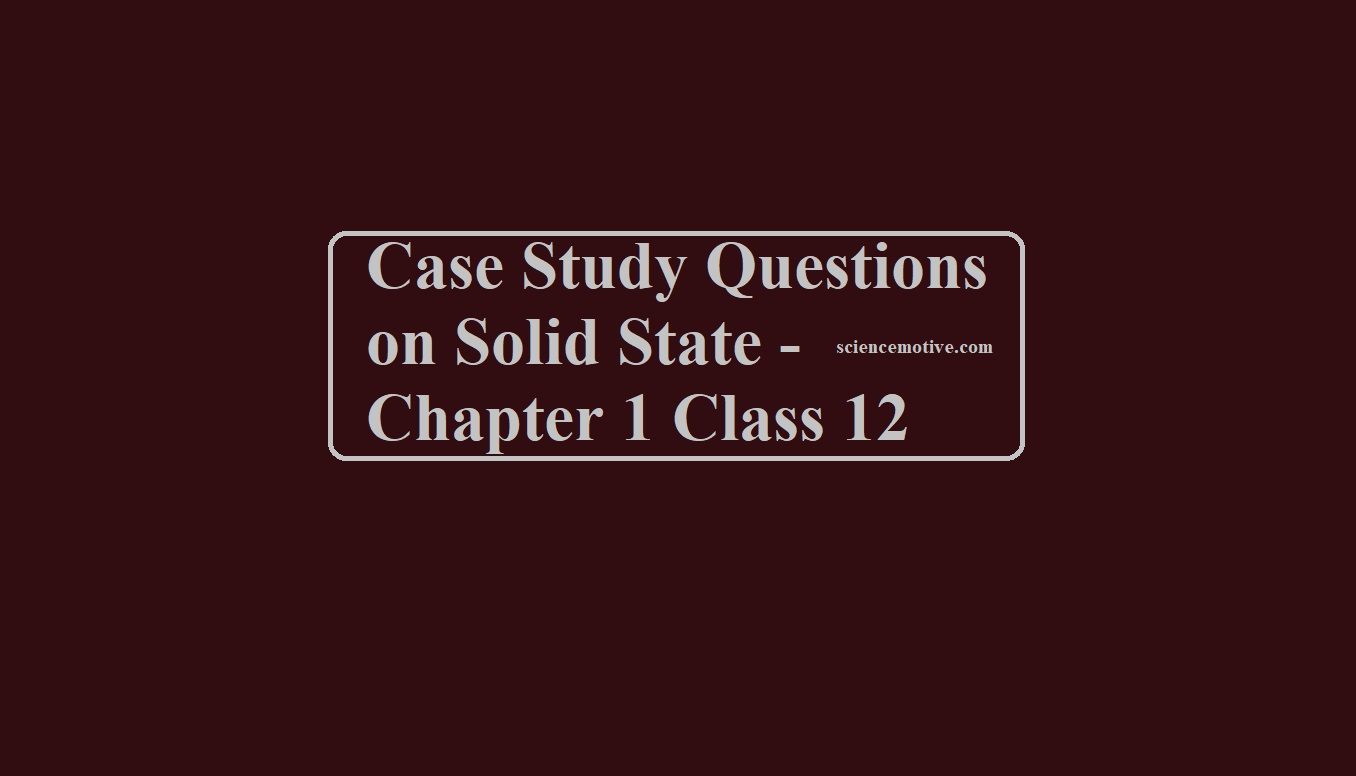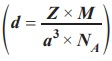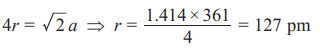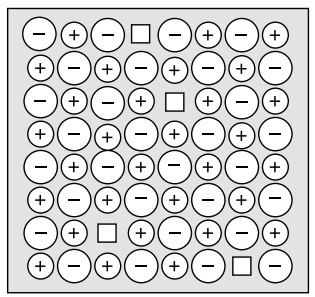# Case Study Questions on Solid State – Chapter 1 Class 12# Case Study Questions on Solid State

All real structures are three-dimensional structures. They can be obtained by stacking two-dimensional layers one above the other while placing the second square close-packed layer above the first we follow the same rule that was followed when one row was placed adjacent to the other. The second layer is placed over the first layer such that the spheres of the upper layer are exactly above there of the first layer. In his arrangement spheres of both the layers are perfectly aligned horizontally as well as vertically. A metallic element crystallizes into a lattice having an ABC ABC pattern and the packing of spheres leaves out voids in the lattice.
Que 1. What type of structure is formed by this arrangement?
(A) ccp                        (B) hcp                        (C) ccp/fcc                  (D) none of the above
Ans 1. (C)
Que 2. Name the non-stoichiometric point defect responsible for colour in alkali metal halides.
(A) Frenkel defect                              (B) Interstitial defect
(C) Schottky defect                             (D) F-centres
Ans 2. (D)
Que 3. What is the total volume of atoms in a face centred cubic unit cell of a metal? (r is the atomic radius).

(A) 16/3 лr3                 (B) лr3                         (C) 24/3 лr3                   (D) 12/3 лr3
Ans 3. (A)
Que 4. Which of the following statements is not true for the amorphous and crystalline solids?
(A) Amorphous solids are isotropic and crystalline solids are anisotropic.
(B) Amorphous solids are short-range order and crystalline solids are long-range order.
(C) Amorphous solids melt at characteristic temperatures while crystalline solids melt over a range of temperatures.
(D) Amorphous solids have irregular shapes and crystalline solids have a geometrical shape.
Ans 4. (C)

Case Study Questions on Solid State

Solids may be classified as amorphous and crystalline. Amorphous solids are isotropic whereas crystalline solids are anisotropic. Solids can also be classified on the basis of type of forces of attraction as ionic, covalent, metallic and molecular solids. Unit cells represent crystal lattice. Types of crystal lattice-bcc, fcc, simple cubic, end centred cubic are most common in seven crystal systems and 14 bravais lattices. The constituent particles arrange in square close packing, bcc, fcc, hcp. The packing efficiency of hcp and fcc is 74%, bcc 68% and simple cubic packing (52%). The vacant sites in packing are called voids, tetrahedral and octahedral being most common. The density of solids can be calculated by usingThere are defects in the crystal lattice, point defects like Frenkel and Schottky. Non-stoichiometric defects are metal excess type and metal deficient type and impurity defects. These defects affect the properties of solids. Solids may be paramagnetic, diamagnetic, ferromagnetic, ferrimagnetic depending upon the presence of unpaired electrons. Solids may be conductors, insulators, semiconductors (n-type and p-type) formed by doping (adding group 13 or 15 as impurity), band theory can explain conductors, semiconductors and insulators.
Que 1. Which type of defects are shown by ZnS?
Ans 1. Frenkel defect.
Que 2. A given metal has an fcc structure with an edge length of 361 pm. What is the radius of an atom?
Ans 2. We knowQue 3. A compound is formed by cation ‘C’ which occupy 75% of octahedral voids and anion ‘A’ form hcp. What is the formula of a compound?
Ans 3. C3A4
Que 4. What is a forbidden zone?
Ans 4. The energy gap between the conduction band and valence band is large in insulators called a forbidden zone.
Que 5. Give one ferrimagnetic mixed oxide.
Ans 5. Fe3O4 is ferrimagnetic.
Que 6. What happens when ZnO is heated and why?
Ans 6. It becomes yellow due to metal excess defect.
Que 7. What type of solid is silicon carbide (Carborundum)?
Ans 7. Covalent solid

Case Study Questions on Solid State

3. Study the diagram given below and answer the following questions:In these questions, a statement of Assertion followed by a statement of Reason is given. Choose the correct answer out of the following choices.
(A) Both A and R are true and R is the correct explanation of A
(B) Both A and R are true but R is NOT the correct explanation of A
(C) A is true but R is false
(D) A is false and R is True
Que 1. Assertion (A): The diagram shows the Schottky defect. Reason
Reason (R): Schottky defect occurs in ionic solids.
Ans 1. (B)
Reason: The diagram shows the Schottky defect as it has an equal number of cationic and anionic vacancies.
Que 2. Assertion (A): LiCl Crystal is pink.
Reason (R): Pink colour of LiCl crystal is due to excess Lithium.
Ans 2. Option (A) is correct.
Reason: LiCl crystal is pink due to excess Lithium. It is caused by a metal excess defect caused by anionic vacancies(F-centres).
Que 3. Assertion (A): The crystal lattice density increases due to the defect shown in the diagram.
Reason (R): Tetrahedral voids are surrounded by 4 constituent particles.
Ans 3. (D)
Reason: The crystal lattice density decreases due to the defect is shown in the diagram-Schottky defect.
Que 4. Assertion (A): AgCl shows Frenkel defect while NaCl does not.
Reason (R): Frenkel defect is shown when anionic vacancies are occupied by unpaired electrons.
Ans 4. (B)
Reason: AgCl shows Frenkel defect while NaCl does not as the size of Ag+ ion is smaller than Na+ ion. Frenkel defect occurs when the smaller ion dislocates from its normal site to an interstitial site.

Case Study Questions on Solid State